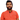# Learn Pain LessHomeOur TeamContact
Python
How to Round in PythonPawneshwer Gupta
March 21, 2023
3 min

01
What is Rounding?
02
Types of Rounding
03
Rounding in Python
04
Conclusion
05
FAQs
06
Final ThoughtsPython is a high-level programming language that provides several built-in functions and methods to perform various mathematical operations. One of the common mathematical operations that programmers need to perform while working on data-related projects is rounding. Rounding refers to the process of reducing the number of decimal places in a number. In this article, we will discuss how to round in Python.

Rounding is the process of reducing the number of decimal places in a number. It is usually done to make the number more manageable or to provide a more simplified representation of the number. For example, if we have a number with many decimal places, we might want to round it off to a certain number of decimal places to make it easier to work with.

## Types of Rounding

There are different types of rounding methods that are commonly used. These include:

This method rounds the number to the nearest integer. If the decimal is 0.5 or greater, the number is rounded up. If the decimal is less than 0.5, the number is rounded down.

### Round Half Down

This method rounds the number to the nearest integer. If the decimal is 0.5 or greater, the number is rounded down. If the decimal is less than 0.5, the number is rounded up.

### Round Half Away from Zero

This method rounds the number to the nearest integer. If the decimal is positive, the number is rounded up. If the decimal is negative, the number is rounded down.

### Round to Even

This method rounds the number to the nearest even integer.

## Rounding in Python

Python provides several built-in functions to perform rounding. Let’s take a look at some of them.

### Round()

The `round()` function rounds a number to a specified number of decimal places. If no decimal places are specified, the function rounds the number to the nearest integer.

`.css-1baulvz{display:inline-block;}num = 3.14159print(round(num)) # Output: 3print(round(num, 2)) # Output: 3.14`

### ceil()

The `ceil()` function rounds a number up to the nearest integer.

```import math
num = 3.14159print(math.ceil(num)) # Output: 4```

### floor()

The `floor()` function rounds a number down to the nearest integer.

```import math
num = 3.14159print(math.floor(num)) # Output: 3```

### trunc()

The `trunc()` function removes the decimal part of a number.

```import math
num = 3.14159print(math.trunc(num)) # Output: 3```

## Conclusion

Rounding is a common mathematical operation that programmers need to perform while working on data-related projects. Python provides several built-in functions to perform rounding, such as `round()`, `ceil()`, `floor()`, and `trunc()`. By understanding these functions, you can perform rounding operations more efficiently in your Python code.

## FAQs

1. What is rounding in Python? Rounding in Python is the process of reducing the number of decimal places in a number.
2. What are the different types of rounding methods? The different types of rounding methods include round half up, round half down, round half away from zero, and round to even.
3. How can I round a number in Python? You can use the built-in round() function in Python to round a number to a specified number of decimal places.
4. How can I round a number up to the nearest integer in Python? You can use the built-in ceil() function in Python to round a number up to the nearest integer.
5. How can I round a number down to the nearest integer in Python? You can use the built-in floor() function in Python to round a number down to the nearest integer.
6. How can I remove the decimal part of a number in Python? You can use the built-in trunc() function in Python to remove the decimal part of a number.
7. Can I specify the number of decimal places while using the ceil() or floor() function in Python? No, the ceil() and floor() functions in Python always round the number up or down to the nearest integer, respectively. If you need to specify the number of decimal places, you should use the round() function instead.
8. Can I use rounding in Python to perform financial calculations? No, rounding in Python should not be used for financial calculations where precision is important. This is because rounding can introduce errors in the calculations. Instead, you should use specialized libraries like Decimal or NumPy for financial calculations.
9. Are there any other rounding functions available in Python? Yes, there are several third-party libraries available in Python that provide additional rounding functions, such as NumPy, Pandas, and Math.

## Final Thoughts

Rounding is a common operation in data-related projects, and Python provides several built-in functions to make it easier to perform rounding. By understanding the different rounding methods and the built-in functions available in Python, you can perform rounding operations efficiently and accurately in your code. However, it’s important to keep in mind that rounding can introduce errors in certain types of calculations, so it should be used with caution.

We'll send you the best of our blog just once a month. We promise.

How To

## Software Developer

Pawneshwer Gupta works as a software engineer who is enthusiastic in creating efficient and innovative software solutions.

Python
Flutter
Laravel
NodeJS

## Related PostsHow to Exit Python Program in Terminal: A Comprehensive Guide
March 23, 2023
3 min